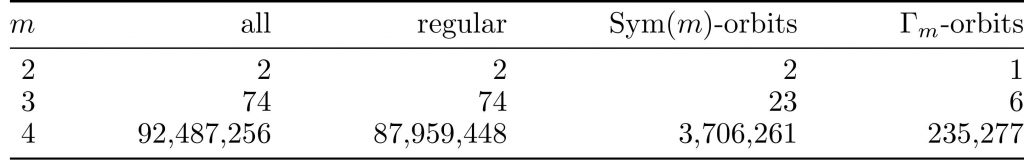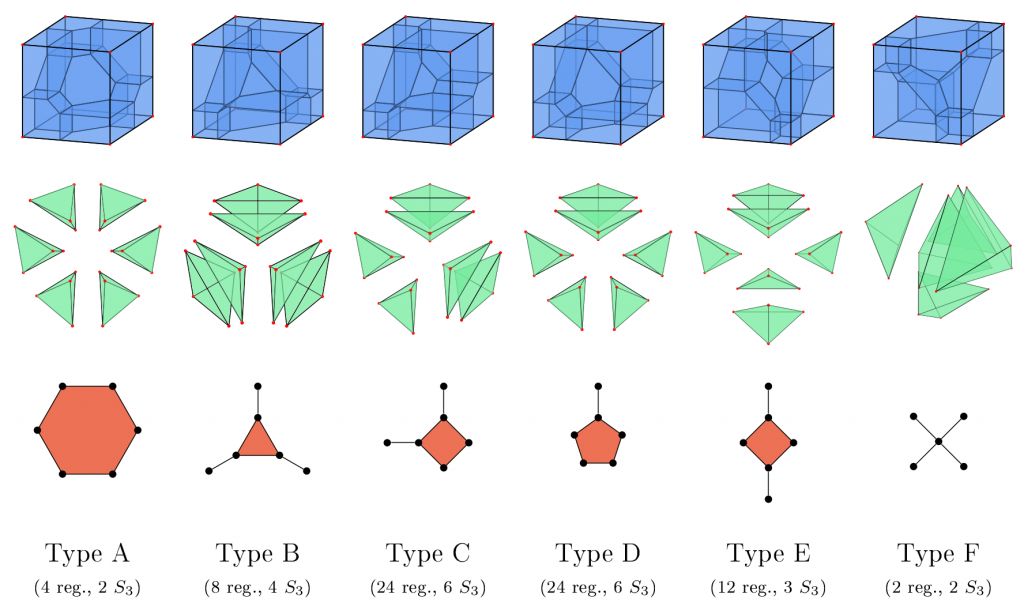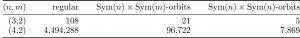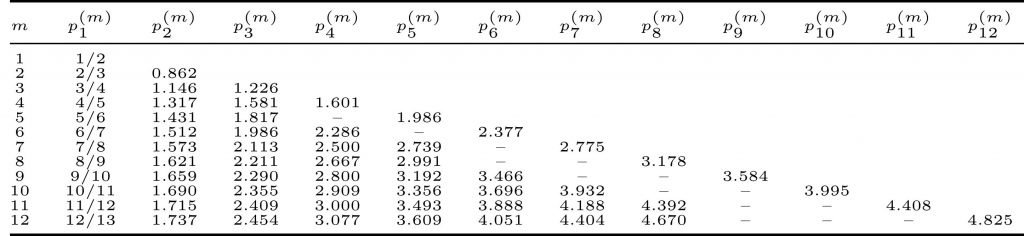# AA3 – Networks

## Tropical Mechanism Design

Project Members

Sylvain Spitz (TU since 01/21, HU until 12/20)

Project Duration

01.01.2019 – 30.09.2022

Located at

TU (since 01/21), HU (until 12/20)

### Description

We study the design of revenue-maximizing mechanisms for selling multiple items. Applying a duality framework, there is a one-to-one correspondence between optimal mechanisms and certain tropical polynomials and rational functions that we want to study via ideas from algebraic statistics.

#### TOPIC AND BACKGROUND OF THE PROJECT

The question of how to sell one item to multiple bidders in a revenue-maximizing auction is well understood since the work of Roger Myerson in the 1980s.  It is used in many applications and on online platforms such as eBay. But if we want to sell multiple items at once, the problem becomes substantially harder and not much is known about the optimal auction mechanism.

#### COMBINATORIAL TYPES OF MECHANISMS

Typically, an auction mechanism asks the participants for their bids and then allocates the items to the bidders as well as a price they have to pay. In order to make the strategy of bidding the true valuation for the items a dominant strategy, it is necessary that the price a bidder has to pay only depends on the bids of all other bidders. This results in a polyhedral subdivision of the valuation space into regions in which the same allocation is chosen by the mechanism.

One way of looking on these regions is to observe how the valuation space of each individual bidder is subdivided, when the bids of the other bidders are fixed. Combinatorially, we can distinguish two subdivisions by studying which regions have a common intersection. In this way, we obtain a correspondence between combinatorial types of mechanisms and regular subdivisions of the cube. We are mainly interested in triangulations up to the symmetries resulting by changing the order of the items. We call the corresponding equivalence classes of triangulations the Sym(m)-orbits, where m stands for the number of items.The following table contains the known number of regular triangulations of the m-cube, together with the Sym(m)-orbits we computed and the Γm-orbits, which count the number of triangulations of the m-cube up to all its symmetries. It follows for example that there are 23 combinatorial types of local mechanisms for 3 items up to permutation.

####The following is a visual representation of the Γ3-orbits for 3 items. The first row represents the subdivision of the valuation space, restricted to valuations in [0,1]3. The second row shows the corresponding triangulation of the cube in an expanded form and the last row shows the tight span of the triangulation which corresponds to the inner cell of the polyhedral subdivision in the first row. The numbers below tell how many regular or Sym(3) triangulations are of the corresponding type.Another way to study these subdivisions is to look for the more global picture. Affine maximizers are a special type of mechanisms which choose the allocation maximizing a weighted social welfare, that is the weighted sum of the bidders’ valuations for the items they get. They can be analyzed in a similar way such that, here as well, we get a correspondence between combinatorial types of affine maximizers and regular subdivisions of products of simplices (instead of the cube). The interesting symmetries now correspond to permuting the items or the bidders (or both), we call them Sym(n)×Sym(m), where n is the number of bidders and m is the number of items. As products of especially two simplices have been studied before, we also include the number of Sym(n)×Sym(n)-orbits which are studied in this subject, although this number does not wear any economic meaning. The numbers for 2 bidders are the same as for the local case before, as all items the first bidder does not get are allocated to the second bidder. For more than 2 bidders, only the numbers for 2 items could be computed.#### SENSITIVITY OF MECHANISMS

An interesting question emerges when we introduce slight perturbations to the bids and wonder how the allocation chosen by the mechanism may change. The allocation can only change from slight perturbations, if the corresponding regions intersect. Thus, we again talk about a combinatorial problem, which we can solve by finding suitable triangulations of the cube (for the local case) or of a product of simplices (for the case of affine maximizers). We want the allocations to not change by much, but first we need to define what we mean by this. In the local case, we like to observe two kinds of distances. The first is just the difference in the amount of items the bidder gets allocated, we call it the cardinality distance. The second counts every item which the bidder either loses or gains, we call it the Hammilton distance. Indeed there is a mechanism guaranteeing that the cardinality distance is always at most one, but for the Hammilton distance we can show that no mechanism can guarantee that it is always less than two. We were only able to prove that there is a mechanism where the Hammilton distance is always less than m-1, where m is the total number of items.

For the case of affine maximizers, we consider the cardinality distance to be the maximum of the cardinality distances of all bidders. One can show that there is a mechanism guaranteeing that the cardinality distance is always at most ⌈m2⌉.

#### MAXIMIZING REVENUE

The mechanisms we discussed this far are called deterministic mechanism, because the items are allocated in a predetermined way. In contrast, we could introduce a lottery for some (or all) items and, after collecting the bids, allocate the items to the bidders up to a certain probability. Observations suggest that in general a revenue-maximizing mechanism has to introduce such lotteries. All the more so it is interesting to find cases, in which a deterministic mechanism is optimal.

Giannakopoulos and Koutsoupias examined the case, where the valuations, that each bidder has for the items, are drawn according to an uniform distribution. They showed, that the optimal mechanism for this setting and up to 6 items is deterministic and they conjectured that this holds true for an arbitrary number of items. We explored their conjecture as well as their approach by applying other methods, especially from tropical geometry.

#### Straight Jacket Auction and Generalized PermutahedraThe deterministic mechanism given by Giannakopoulos and Koutsoupias is called the Straight Jacket Auction (SJA). It consists of a certain price schedule (p1,…,pm), where pk designates the price for any bundle of k items. As discussed before, such a price schedule divides the m-cube in regions depending on the term which maximizes the bidders’ utility function u(x) = max{ Σj∈J x– p|J] | J ⊂ {1,…,m} }. The regions are marked on the right by UJ, where J is the bundle of items that attain the maximum utility. Their volumetric properties are essential for the computation of the prices and the proof of optimality of the mechanism.

The regions are in fact covector cells of the tropical polynomial u(x) intersected with the m-cube. They can also be classified as (products of) generalized permutahedra, which is useful since those polytopes come up in a variety of applications and are therefore well studied. This way, we are able to derive multiple volume formulae for the regions, which link them to a counting problem about the number of certain bipartite graphs, Delzant polytopes and Lorentzian polynomials.

The SJA-prices are known to be optimal for up to 6 items and conjectured to be optimal in general. We contribute to this conjecture by showing that the prices indeed satisfy some necessary optimality conditions and that there are no other symmetric and strictly submodular price schedules satisfying those conditions.

#### Computation of SJA-prices

The prices for the Straight-Jacket-Auction are not easy to compute. We used our insights to implement an algorithm using HomotopyContinuation.jl to calculate the prices for up to 12 items (before known for up to 6).Selected Publications

• Michael Joswig and Max Klimm and Sylvain Spitz, The polyhedral geometry of truthful auctions, arxiv:2211.01907, 2022.
• Michael Joswig and Max Klimm and Sylvain Spitz, Generalized permutahedra and optimal auctions, SIAM J. Appl. Algebra Geom., arxiv:2108.00979, to appear.
• Michael Joswig, The Cayley trick for tropical hyper surfaces with a view toward Ricardian economics, Homological and computational methods in commutative algebra, 107-128, 2017.
• Paul Dütting and Felix Fischer and Max Klimm, Revenue Gaps for Static and Dynamic Posted Pricing of Homogenous Goods, arXiv:1607.07105, 2019.

Poster

Please insert any kind of pictures (photos, diagramms, simulations, graphics) related to the project in the above right field (Image with Text), by choosing the green plus image on top of the text editor. (You will be directed to the media library where you can add new files.)
(We need pictures for a lot of purposes in different contexts, like posters, scientific reports, flyers, website,…
Please upload pictures that might be just nice to look at, illustrate, explain or summarize your work.)